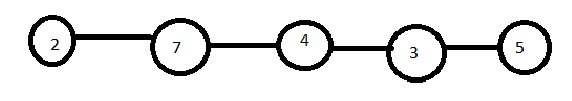# Finding next greater node for each node in JavaScript

## Problem

We are required to write a JavaScript function that takes in the head of the linked list as the first and the only argument.

This linkedlist contains numerical data. Each node in the list may have a next larger value: for node_i, next_larger(node_i) is the node_j.val such that j > i, node_j.val > node_i.val, and j is the smallest possible choice. If such a j does not exist, the next larger value is 0.

Our function should prepare and return an array in which the corresponding element is the next greater element for the element in the list.

For example, if the list is −Then the output should be −

const output = [7, 0, 5, 5, 0];

## Output Explanation:

Because the next greater element of 2 is 7, for 7 there is no greater element and so on.

## Example

The code for this will be −

Live Demo

class Node{
constructor(data){
this.data = data;
this.next = null;
};
};
constructor(){
this.size = 0;
};
};
const newNode = new Node(data);
let curr
}else{
while (curr.next) {
curr = curr.next;
}
curr.next = newNode;
};
this.size++;
};
const nextGreater = (head) => {
const arr = [];
const res = [];
let currentIndex = 0
while(curr){
while (arr.length > 0 && curr.data > arr[arr.length - 1]) {
const [index] = arr.pop();
res[index] = curr.data;
};
arr.push([currentIndex, curr.data]);
currentIndex += 1;
curr = curr.next;
};
for(let i = 0; i < currentIndex; i++){
if(res[i] === undefined){
res[i] = 0;
};
};
return res;
};
console.log(nextGreater(list.head));

## Output

And the output in the console will be −

[ 7, 0, 5, 5, 0 ]